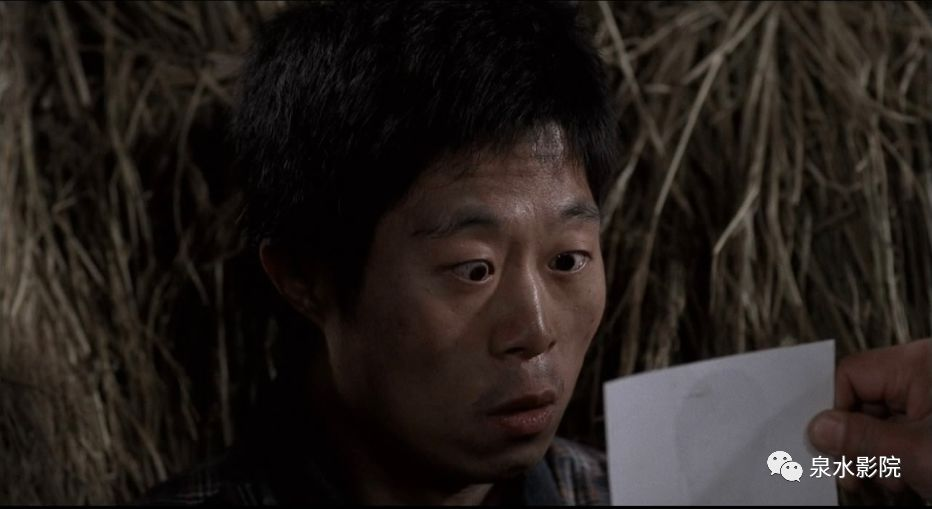﻿ 有句话是事实不能大白于天下——TOP纪实悬疑【杀人回忆】 - 永州新闻网

# 有句话是事实不能大白于天下——TOP纪实悬疑【杀人回忆】

《杀人回忆》 被许多观众誉为韩国悬疑电影史上的No.1，导演正是刚刚在戛纳电影节凭 《寄生虫》 拿了金棕榈大奖的奉俊昊。2019年9月25日，在案件过去29年之余，真凶终于落网，并且被韩国媒体公布了姓名。天网恢恢疏而不漏，小泉首先希望被害人安息，而当时办案的警察也终于能放下困扰心头多年的梦魇。' style='' data-lazy='1' data-height='602' data-width='900' width='900' height='auto'>' style='' data-lazy='1' data-height='483' data-width='900' width='900' height='auto'>' style='' data-lazy='1' data-height='491' data-width='900' width='900' height='auto'>' style='' data-lazy='1' data-height='398' data-width='548' width='548' height='auto'>' style='' data-lazy='1' data-height='503' data-width='900' width='900' height='auto'>' style='' data-lazy='1' data-height='157' data-width='280' width='280' height='auto'>' style='' data-lazy='1' data-height='527' data-width='795' width='795' height='auto'>' style='' data-lazy='1' data-height='442' data-width='675' width='675' height='auto'>' style='' data-lazy='1' data-height='492' data-width='900' width='900' height='auto'>' style='' data-lazy='1' data-height='582' data-width='469' width='469' height='auto'>' style='' data-lazy='1' data-height='402' data-width='553' width='553' height='auto'>' style='' data-lazy='1' data-height='394' data-width='546' width='546' height='auto'>' style='' data-lazy='1' data-height='479' data-width='838' width='838' height='auto'>' style='' data-lazy='1' data-height='486' data-width='707' width='707' height='auto'>' style='' data-lazy='1' data-height='488' data-width='900' width='900' height='auto'>' style='' data-lazy='1' data-height='507' data-width='900' width='900' height='auto'>' style='' data-lazy='1' data-height='217' data-width='400' width='400' height='auto'>' style='' data-lazy='1' data-height='505' data-width='900' width='900' height='auto'>' style='' data-lazy='1' data-height='416' data-width='765' width='765' height='auto'>' style='' data-lazy='1' data-height='576' data-width='900' width='900' height='auto'>' style='' data-lazy='1' data-height='544' data-width='900' width='900' height='auto'>' style='' data-lazy='1' data-height='547' data-width='900' width='900' height='auto'>' style='' data-lazy='1' data-height='489' data-width='900' width='900' height='auto'>' style='' data-lazy='1' data-height='544' data-width='900' width='900' height='auto'>' style='' data-lazy='1' data-height='485' data-width='900' width='900' height='auto'>' style='' data-lazy='1' data-height='396' data-width='546' width='546' height='auto'>' style='' data-lazy='1' data-height='827' data-width='523' width='523' height='auto'>

-